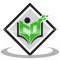## Half of a herd of deer are grazing in the field and three fourths of the remaining are playing nearby. The rest 9 are drinking water from the pond. Find the number of deer in the herd.Updated on 10-Oct-2022 13:47:38

## The organisers of an essay competition decide that a winner in the competition gets a prize of $Rs. 100$ and a participant who does not win gets a prize of $Rs. 25$. The total prize money distributed is Rs. 3,000 . Find the number of winners, if the total number of participants is $63 .$Updated on 10-Oct-2022 13:47:38
Given :A winner in the competition gets a prize of Rs 100 and a participant who does not win gets a prize of Rs 25. The total prize money distributed is Rs 3,000.The total number of participants $= 63$.To find :We have to find the number of winners.Solution :Let the number of winners be $x$.This implies, that the number of people who did not win $= 63 - x$Therefore,$Rs. x(100)+ Rs. (63-x)(25) = Rs. 3000$$100x + 63(25) -25x = 3000$$(100-25)x + 1575 = 3000$$75x = 3000 - 1575$$75x = 1425$$x=\frac{1425}{75}$$x=\frac{57}{3}$$x=19The number of winners is 19. ## I have a total of $Rs. 300$ in coins of denomination $Rs. 1, Rs. 2$ and $Rs. 5$. The number of $Rs. 2$ coins is 3 times the number of $Rs. 5$ coins. The total number of coins is 160 . How many coins of each denomination are with me?Updated on 10-Oct-2022 13:47:38 Given :The total number of coins = 160.Sum total of all coins = Rs. 300.Coins are in the denomination \ Rs. 1, \ Rs. 2, and \ Rs. 5. The number of \ Rs. 2 coins is 3 times the number of \ Rs. 5 coins.To do :We have to find the number of coins in each denomination.Solution :Let the number of Rs. 1 coins be x and the number of Rs. 5 coins be y.The number of Rs. 2 coins is 3 times the number of Rs. 5 coins.This implies, The number of Rs. 2 coins = 3y.Therefore, x+3y+y=160$$x+4y=160$.................(i)$[x(1)+3y(2)+y(5)] ... Read More ## Baichung's father is 26 years younger than Baichung's grandfather and 29 years older than Baichung. The sum of the ages of all the three is 135 years. What is the age of each one of them?Updated on 10-Oct-2022 13:47:38 Given:Baichung's Father is 26 years younger than Baichung's Grandfather and 29 years older than Baichung.The sum of the ages of all the three is 135. To do :We have to find the ages of each of them.Solution :Let the age of Baichung be$x$and the age of the grandfather be$y$.This implies, Age of Baichung's father$= x+29 = y-26$$y = x+29+26 = x+55The sum of the ages of all the three = 135$$x+(x+29)+y = 135$$x+x+29+x+55 = 135$$3x+84 = 135$$3x = 135-84$$3x = 51$$x = \frac{51}{3}$$x = 17$$x+29 = 17+29=46$$y = x+55 = 17+55=72$The age of Baichung is 17 years.The ... Read More ## The number of boys and girls in a class are in the ratio 7:5. The number of boys is 8 more than the number of girls. Whar is the total class strength?Updated on 10-Oct-2022 13:47:38 Given:The number of boys and girls are in the ratio of$7:5$.The number of boys is 8 more than the number of girls.To do:We have to find the total strength of the class.Solution:Let the number of boys be$7x$and the number of girls be$5x$.According to the question,$7x = 5x+8$$7x-5x=8$$2x=8$$x=\frac{8}{2}$$x=4$Therefore,The number of boys$= 7x = 7(4) = 28$.The number of girls$= 5x = 5(4) =20$.The total strength of the class$= 28+20 = 48$.Therefore, the total number of students in the class is$48$. ## Three consecutive integers are such that when they are taken in increasing order and multiplied by 2,3 and 4 respectively, they add up to 74 . Find these numbers.Updated on 10-Oct-2022 13:47:38 Given: Three consecutive integers are such that when they are taken in increasing order and multiplied by 2,3 and 4 respectively, they add up to 74.To do:We have to find the numbers.Solution:Let the three consecutive integers be$x, x+1$and$x+2$According to the given question,$2\times x+ 3\times(x+1) + 4\times(x+2) = 74$$2x + 3x + 3 + 4x + 8 = 74$$9x+11=74$$9x = 74-11$$9x = 63$$x = \frac{63}{9}$$x = 7$$\Rightarrow x+1=7+1=8$$x+2 = 7+2 =  9$Therefore, the required numbers are$7, 8$and$9$. ## Three consecutive integers add up to 51 . What are these integers?Updated on 10-Oct-2022 13:47:38 Given:Three consecutive integers add up to 51.To do:We have to find the integers.Solution:Let the smallest integer be$x$.This implies,The three consecutive integers are$x, x+1, x+2$.According to the question,$x + x + 1 + x + 2 = 51$$3x + 3 = 51$$3x = 51-3$$3x = 48$$x = \frac{48}{3}$$x = 16So, the numbers arex = 16$$x + 1 = 16 + 1 = 17x + 2 = 16 + 2 = 18$The required integers are$16, 17$and$18\$.
Previous 1 ... 5 6 7 8 9 ... 2384 Next